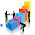## 30 Jan 2017

Q.1 The main port of gujrat is
Ans. Kandla
Q.2 The book Das Capital has been written by-
Ans. Karl Marks
Q.3 The Great Victoria desert is located in-
Ans. Australia
Q.4 Nagar Uday Abhiyan has been launched by-
Q.5 Rashtriya Ekta Diwas  is observed on-
Ans. 31st October
Q.6 The Salt Satyagrah started on-
Ans. 12 March, 1930
Q.7 Find the odd one-
2, 5, 10, 26, 37, 50, 64
Ans. 64
Q.8 If TABLE:UBCMF::CHAIR:?
Ans. DIBJS
Q.9 What is the 25th digit to the right of the decimal point in the decimal form of 6/11?
Ans. 5
Q.10 A carpenter constructed a rectangular frame with area of 10m2. If the carpenter were to make similar frame twice as long and twice as wide as the first frame, what would be the area of second frame?
Ans. 40#

Q.11 Find the odd one-
Ans. AC
Q.12 If in a coding language word SMEAL= *+#@&, then what would be ALELSME=?
Ans. D- @&#*+#
Q.13 Let N be the greatest number that will divide 1305, 4665 and 6905, leaving the same reminder in each case. Then sum of the digit in N is ?
Ans. 4

Q.14 On selling 17 balls at Rs. 720, there is a loss equal to the cost price of 5 balls. The cost price of a ball is
Ans. 60
Q.15 There are two examinations rooms A and B. If 10 students are sent from A to B, then the number of students in each room is the same. If 20 candidates are sent from B to A, then the number of students in A is double the number of students in B. The number of students in room A is
Ans. 100

1.where are rest of the que ans, paper comprised of 80 questions.

2.3.4.Wait upto 8.00 PM

5.Que 10 Ans wrong . Check again rect frame given not a box and area is in metre square. Ans is 40

6.Que 10 Ans wrong . Check again rect frame given not a box and area is in metre square. Ans is 40

7.Is test ki genral catagry k liye approx cut of list kya ho sakti hai

8.1.9.10.